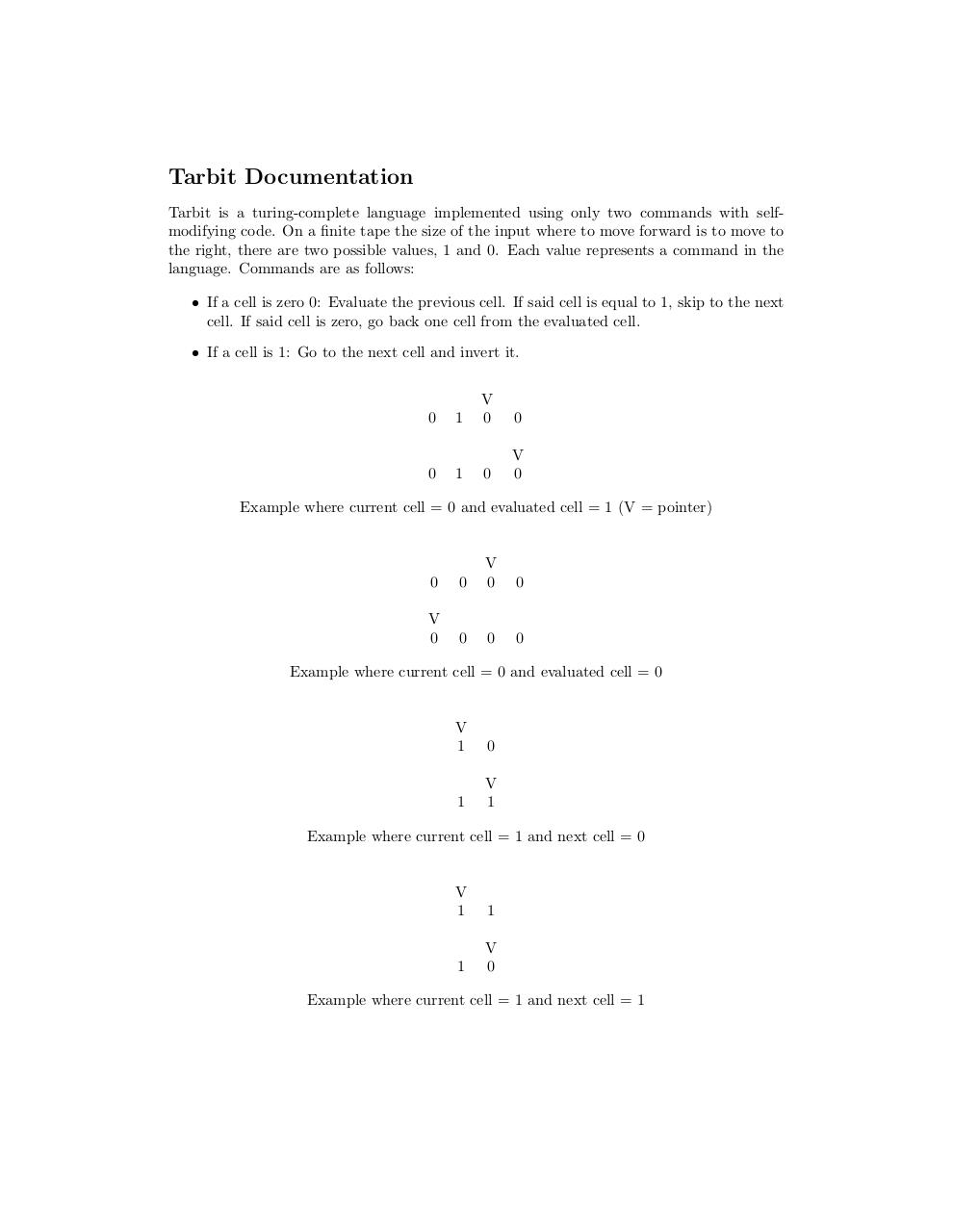# tarbit docs .pdf

### File information

Original filename: tarbit docs.pdf

This PDF 1.5 document has been generated by TeX / MiKTeX pdfTeX-1.40.18, and has been sent on pdf-archive.com on 06/10/2017 at 01:33, from IP address 104.220.x.x. The current document download page has been viewed 158 times.
File size: 36 KB (1 page).
Privacy: public file

tarbit docs.pdf (PDF, 36 KB)

### Document preview

Tarbit Documentation
Tarbit is a turing-complete language implemented using only two commands with selfmodifying code. On a finite tape the size of the input where to move forward is to move to
the right, there are two possible values, 1 and 0. Each value represents a command in the
language. Commands are as follows:
• If a cell is zero 0: Evaluate the previous cell. If said cell is equal to 1, skip to the next
cell. If said cell is zero, go back one cell from the evaluated cell.
• If a cell is 1: Go to the next cell and invert it.

0

1

V
0

0

0

1

0

V
0

Example where current cell = 0 and evaluated cell = 1 (V = pointer)

0

0

V
0

0

V
0

0

0

0

Example where current cell = 0 and evaluated cell = 0

V
1

0

1

V
1

Example where current cell = 1 and next cell = 0

V
1

1

1

V
0

Example where current cell = 1 and next cell = 1#### HTML Code

Copy the following HTML code to share your document on a Website or Blog

#### QR Code### Related keywords